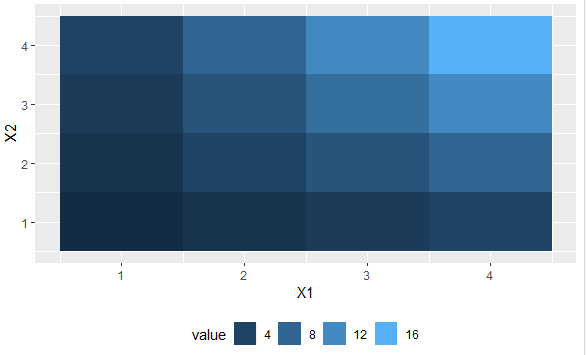# ggplot2 legend to bottom and horizontal

1 view

How can I move a ggplot2 legend to the bottom of the plot and turn it horizontally?

Sample code:

library(reshape2) # for melt

df <- melt(outer(1:4, 1:4), varnames = c("X1", "X2"))

p1 <- ggplot(df, aes(X1, X2)) + geom_tile(aes(fill = value))

p1 + scale_fill_continuous(guide = guide_legend())by (25.3k points)

To move the legend to the bottom, use the following code:

library(reshape2) # for melt

df <- melt(outer(1:4, 1:4), varnames = c("X1", "X2"))

p1 <- ggplot(df, aes(X1, X2)) + geom_tile(aes(fill = value))

p1 + scale_fill_continuous(guide = guide_legend()) +

theme(legend.position="bottom")

Output: